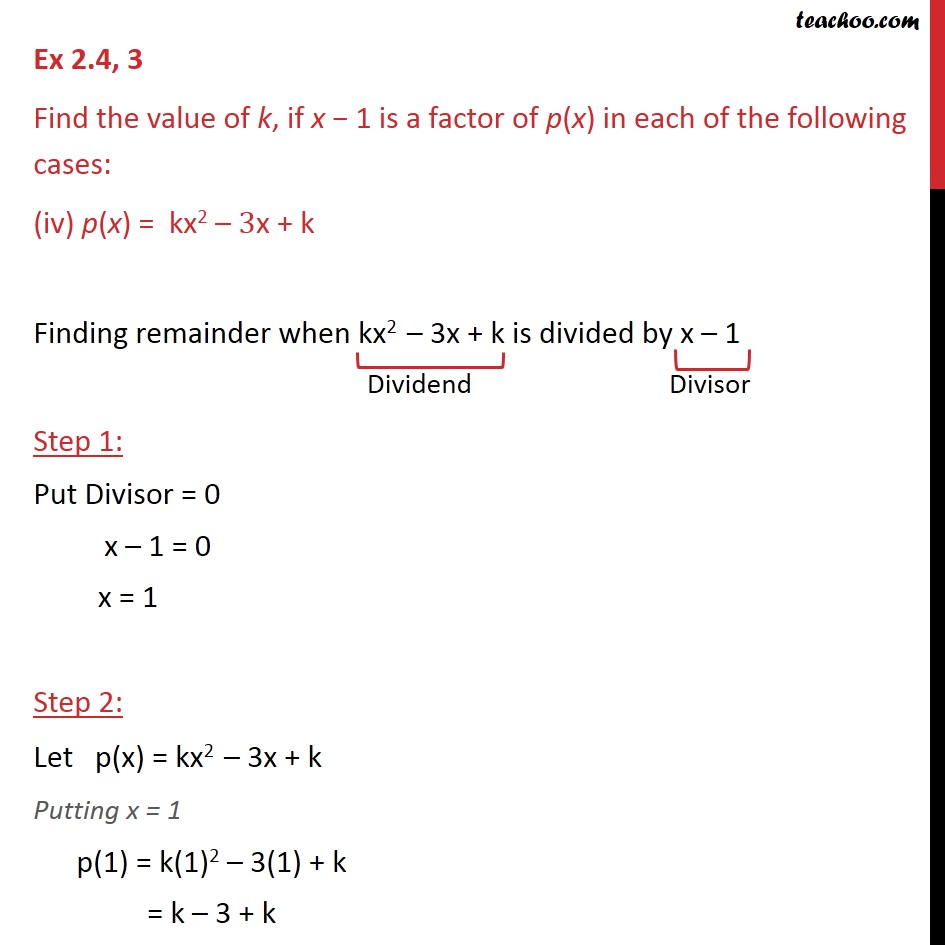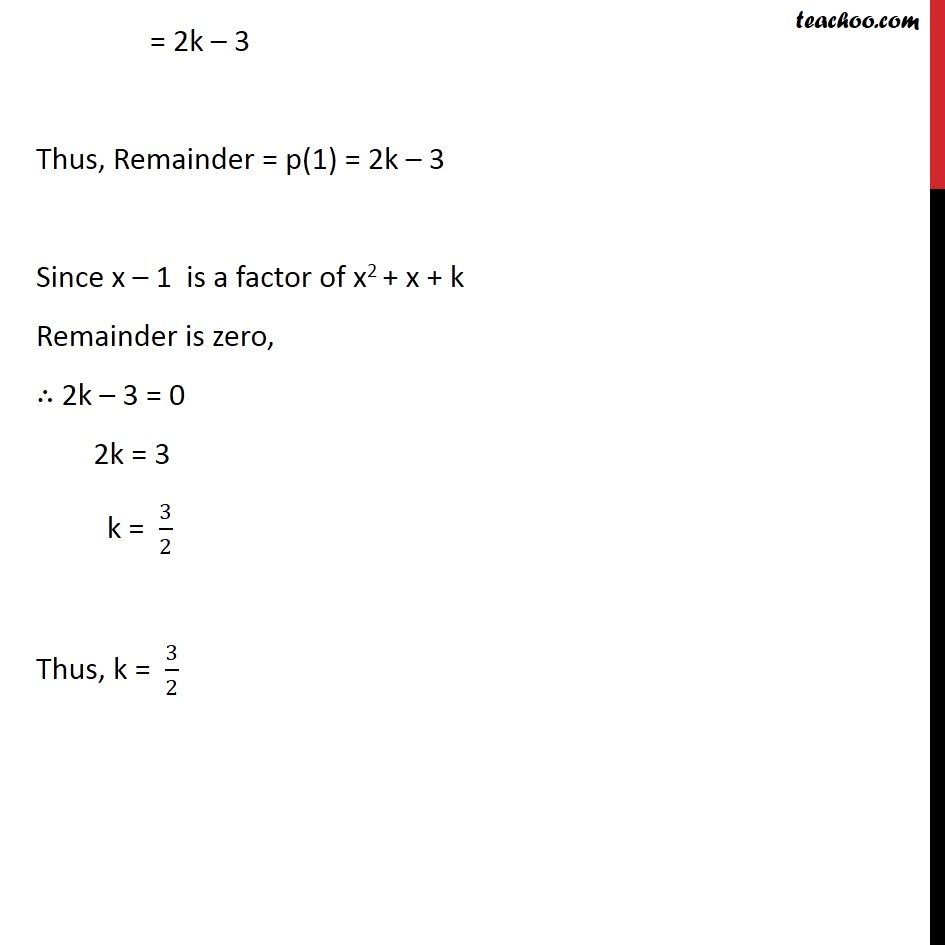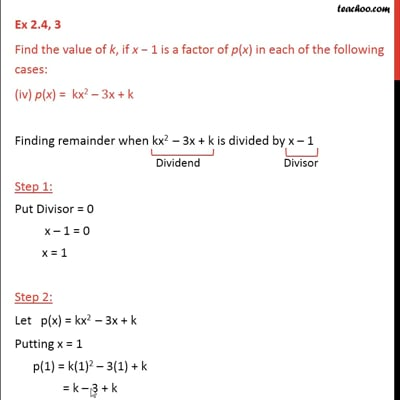Ex 2.4

Chapter 2 Class 9 Polynomials
Serial order wiseThis video is only available for Teachoo black users

Introducing your new favourite teacher - Teachoo Black, at only ₹83 per month

### Transcript

Ex 2.4, 3 Find the value of k, if x 1 is a factor of p(x) in each of the following cases: (iv) p(x) = kx2 3x + k Finding remainder when kx2 3x + k is divided by x 1 Step 1: Put Divisor = 0 x 1 = 0 x = 1 Step 2: Let p(x) = kx2 3x + k Putting x = 1 p(1) = k(1)2 3(1) + k = k 3 + k = 2k 3 Thus, Remainder = p(1) = 2k 3 Since x 1 is a factor of x2 + x + k Remainder is zero, 2k 3 = 0 2k = 3 k = 3/2 Thus, k = 3/2